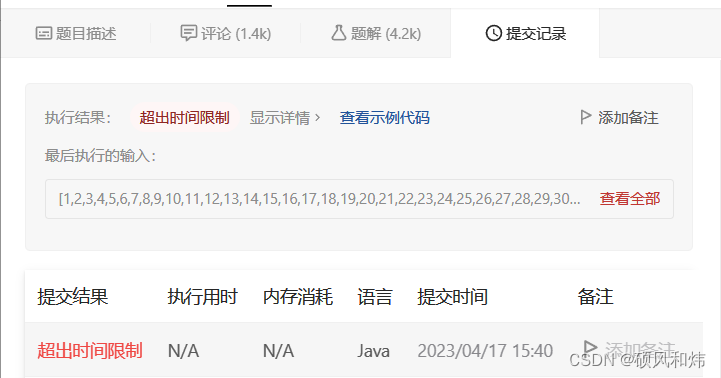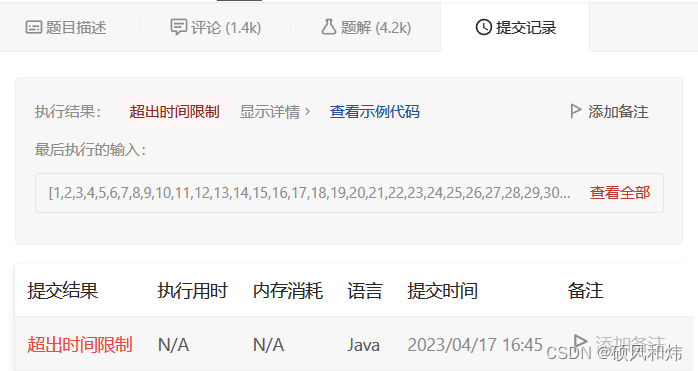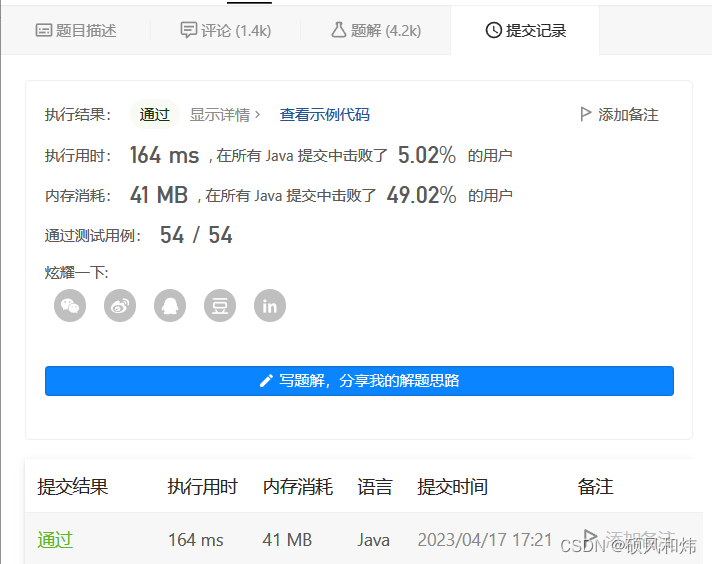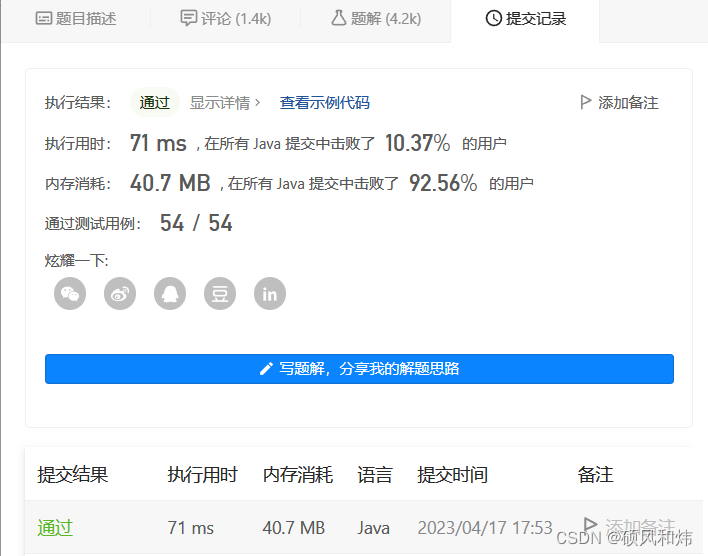# 【LeetCode: 300. 最长递增子序列 | 暴力递归=＞记忆化搜索=＞动态规划】🚀 算法题 🚀

🌲 算法刷题专栏 | 面试必备算法 | 面试高频算法 🍀
🌲 越难的东西,越要努力坚持，因为它具有很高的价值，算法就是这样✨
🌲 作者简介：硕风和炜，CSDN-Java领域新星创作者🏆，保研|国家奖学金|高中学习JAVA|大学完善JAVA开发技术栈|面试刷题|面经八股文|经验分享|好用的网站工具分享💎💎💎
🌲 恭喜你发现一枚宝藏博主,赶快收入囊中吧🌻
🌲 人生如棋，我愿为卒，行动虽慢，可谁曾见我后退一步？🎯🎯

 🚀 算法题 🚀### 🍔 目录

• 🚩 题目链接
• ⛲ 题目描述
• 🌟 求解思路&实现代码&运行结果
• ⚡ 暴力递归1
• 🥦 求解思路
• 🥦 实现代码
• 🥦 运行结果
• ⚡ 暴力递归2
• 🥦 求解思路
• 🥦 实现代码
• 🥦 运行结果
• ⚡ 记忆化搜索
• 🥦 求解思路
• 🥦 实现代码
• 🥦 运行结果
• ⚡ 动态规划
• 🥦 求解思路
• 🥦 实现代码
• 🥦 运行结果
• ⚡ 动态规划 + 二分查找
• 🥦 求解思路
• 🥦 实现代码
• 🥦 运行结果
• 💬 共勉

• 300. 最长递增子序列

## ⛲ 题目描述

1 <= nums.length <= 2500
-104 <= nums[i] <= 104

## 🌟 求解思路&实现代码&运行结果

### ⚡ 暴力递归1

#### 🥦 求解思路

1. 根据题目的意思，让我们求得最长递增子序列的长度是什么，我们可以设计这样一个递归函数，从某一个位置开始，如果当前选择的数字是大于我们之前选择的数字的，那么我们记录长度的变量++，否则，进行下一个位置的判断，之前选择的数不变，记录长度的也不变。
2. 接下来我们来看一下具体代码的实现。

#### 🥦 实现代码

``````class Solution {

public int lengthOfLIS(int[] nums) {
return process(0,nums,0,Integer.MIN_VALUE);
}

public int process(int index,int[] nums,int cnt,int pre){
if(index==nums.length){
return cnt;
}
int max=Integer.MIN_VALUE;
for(int i=index;i<nums.length;i++){
if(nums[i]>pre){
max=Math.max(max,process(i+1,nums,cnt+1,nums[i]));
}else{
max=Math.max(max,process(i+1,nums,cnt,pre));
}
}
return max;
}
}
``````

#### 🥦 运行结果### ⚡ 暴力递归2

#### 🥦 求解思路

1. 上面虽然实现了一个递归版本的题解，时间超限了，是我们期待的结果，但是并不是一个好的递归版本，因为状态参数太多了，不方便都维护。
2. 接下来我们就再来想一想，还有没有可能设计一个更好的递归函数呢？
3. 我们可以设计这样一个递归函数，以当前index位置为结尾，递归函数中每次从0到index-1的位置进行选择，如果存在元素小于当前index位置的元素，继续递归调用，下一个位置从当前小于index位置开始，重复该过程，直至结束。
4. 我们可以看到，这个递归函数比起我们上面设计的递归函数精简不少，接下来我们就来一起看一下具体的实现。

#### 🥦 实现代码

``````class Solution {

public int lengthOfLIS(int[] nums) {
int max=Integer.MIN_VALUE;
for(int i=0;i<nums.length;i++){
max=Math.max(max,process(i,nums));
}
return max;
}

public int process(int index,int[] nums){
if(index==nums.length){
return 0;
}
int max=1;
for(int i=0;i<index;i++){
if(nums[i]<nums[index]){
max=Math.max(max,process(i,nums)+1);
}
}
return max;
}
}
``````

#### 🥦 运行结果### ⚡ 记忆化搜索

#### 🥦 求解思路

1. 根据我们递归的分析，在递归的过程中会产生重复的子过程，所以我们想到了加一个缓存表，也就是我们的记忆化搜索。

#### 🥦 实现代码

``````class Solution {

public int lengthOfLIS(int[] nums) {
int max=Integer.MIN_VALUE;
int n=nums.length;
int[] dp=new int[n];
Arrays.fill(dp,1);
for(int i=0;i<n;i++){
max=Math.max(max,process(i,nums,dp));
}
return max;
}

public int process(int index,int[] nums,int[] dp){
if(index==nums.length){
return 0;
}
if(dp[index]!=1) return dp[index];
int max=1;
for(int i=index;i<nums.length;i++){
if(nums[i]>nums[index]){
max=Math.max(max,process(i,nums,dp)+1);
}
}
return dp[index]=max;
}
}
``````

#### 🥦 运行结果### ⚡ 动态规划

#### 🥦 求解思路

1. 按照我们之前递归和记忆化搜索的思路，通过动态规划实现出来。

#### 🥦 实现代码

``````class Solution {

public int lengthOfLIS(int[] nums) {
int n=nums.length;
int[] dp=new int[n];
int max=0;
Arrays.fill(dp,1);
for(int index=n-1;index>=0;index--){
for(int i=index;i<n;i++){
if(nums[i]>nums[index]){
dp[index]=Math.max(dp[index],dp[i]+1);
}
}
max=Math.max(max,dp[index]);
}
return max;
}
}
``````

#### 🥦 运行结果### ⚡ 动态规划 + 二分查找

#### 🥦 求解思路

1. 因为题目要我们查找的是递增子序列，也就是元素按照严格递增排列的，所以我们可以想到使用二分来进行优化。
2. 我们将每次查找的结果放到开辟的数组空间中，具体怎么做呢？
3. 我们可以每次遍历我们nums中的数字x，然后在收集数组的区间内进行查找大于当前x做左侧的位置，最后将该位置设置为x；
4. 如果此时查找的边界到达了我们此时数组下标位置，说明此时该元素是大于数组中所有元素的，此时数组下标位置继续向右移动。

#### 🥦 实现代码

``````class Solution {
public int lengthOfLIS(int[] nums) {
int[] dp = new int[nums.length];
int res = 0;
for (int x : nums) {
int left = -1, right = res;
while(left+1 < right){
int mid = left + ((right - left) >> 1);
if(dp[mid] < x){
left = mid;
}else{
right = mid;
}
}
dp[right] = x;
if(right == res){
res++;
}
}
return res;
}
}
``````

#### 🥦 运行结果## 💬 共勉